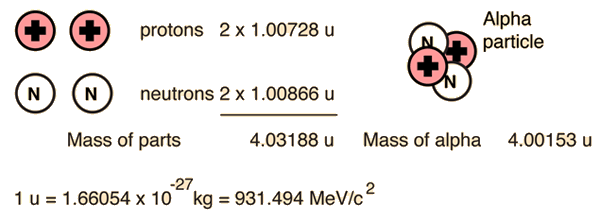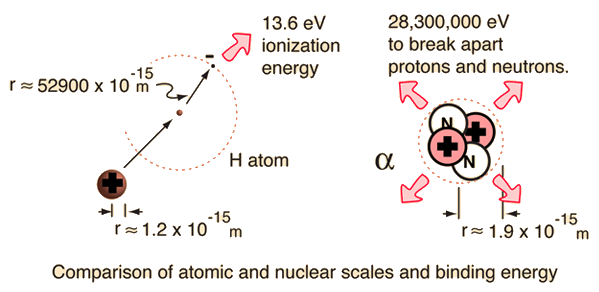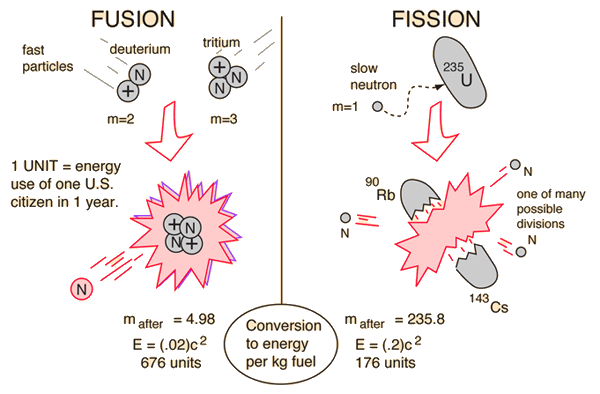# Nuclear Binding Energy

Nuclei are made up of protons and neutrons, but the mass of a nucleus is always less than the sum of the individual masses of the protons and neutrons which constitute it. The difference is a measure of the nuclear binding energy which holds the nucleus together. This binding energy can be calculated from the Einstein relationship:

Nuclear binding energy = Δmc2

For the alpha particle Δm= 0.0304 u which gives a binding energy of 28.3 MeV.The enormity of the nuclear binding energy can perhaps be better appreciated by comparing it to the binding energy of an electron in an atom. The comparison of the alpha particle binding energy with the binding energy of the electron in a hydrogen atom is shown below. The nuclear binding energies are on the order of a million times greater than the electron binding energies of atoms.Binding energy curve. Nuclear units.
Index

Nuclear Structure Concepts

 HyperPhysics***** Nuclear R Nave
Go Back

# Fission and fusion can yield energyNuclear binding energy Fusion example Fission example Further discussion
Index

Nuclear fission concepts

Nuclear fusion concepts

 HyperPhysics***** Nuclear R Nave
Go Back

# Nuclear Binding Energy Curve

The binding energy curve is obtained by dividing the total nuclear binding energy by the number of nucleons. The fact that there is a peak in the binding energy curve in the region of stability near iron means that either the breakup of heavier nuclei (fission) or the combining of lighter nuclei (fusion) will yield nuclei which are more tightly bound (less mass per nucleon).

The binding energies of nucleons are in the range of millions of electron volts compared to tens of eV for atomic electrons. Whereas an atomic transition might emit a photon in the range of a few electron volts, perhaps in the visible light region, nuclear transitions can emit gamma-rays with quantum energies in the MeV range.

### The iron limit

The buildup of heavier elements in the nuclear fusion processes in stars is limited to elements below iron, since the fusion of iron would subtract energy rather than provide it. Iron-56 is abundant in stellar processes, and with a binding energy per nucleon of 8.8 MeV, it is the third most tightly bound of the nuclides. Its average binding energy per nucleon is exceeded only by 58Fe and 62Ni, the nickel isotope being the most tightly bound of the nuclides.
 Nuclear binding energy curve Nuclear binding energy Fusion example Fission example
 The most tightly bound nuclei
Index

Nuclear fission concepts

Nuclear fusion concepts

 HyperPhysics***** Nuclear R Nave
Go Back

# Fission and Fusion YieldsDeuterium-tritium fusion and uranium-235 fission are compared in terms of energy yield. Both the single event energy and the energy per kilogram of fuel are compared. Then they are expressed in terms of a nominal per capita U.S. energy use: 5 x 1011 joules. This figure is dated and probably high, but it gives a basis for comparison. The values above are the total energy yield, not the energy delivered to a consumer.

 Illustration Comparison unit:1 U.S. year Further discussion
 Fission fragments
Index

Nuclear fission concepts

Nuclear fusion concepts

 HyperPhysics***** Nuclear R Nave
Go BackEnergy comparison Further discussion Fission fragments
Index

Nuclear fission concepts

Nuclear fusion concepts

 HyperPhysics***** Nuclear R Nave
Go Back